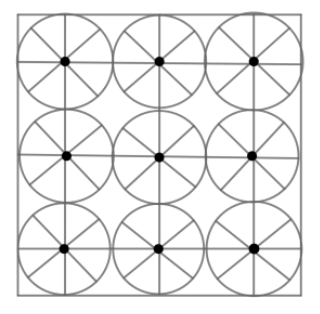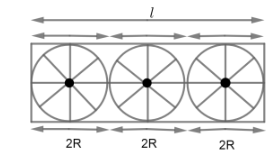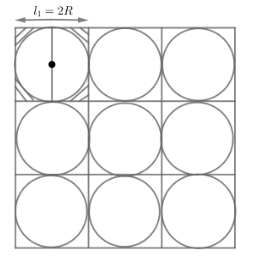QUESTION

# On a square handkerchief, nine circular designs each of radius 7am are made. Find the area of the remaining portion of the handkerchief.Hint: In the given diagram, there are two types of geometrical shapes whose formula of area is known to us – circle and square. So, for finding the remaining area we have to find the area of the square and area covered by all circular designs and finally subtract the area covered by circular designs from the total area of the square to get the required area. Radius of the circle is given in question and the side of the square can be calculated by observing the given diagram.

We know, area of square $={{\left( side \right)}^{2}}$
Handkerchief is of square shape.
So, total area of the handkerchief $={{\left( \text{side}\ \text{length}\ \text{of}\ \text{handkerchief} \right)}^{2}}........\left( 1 \right)$
According to question,From the diagram above, we can observe that
\begin{align} & l=\left( 2R \right)+\left( 2R \right)+\left( 2R \right) \\ & \Rightarrow l=6R.......................\left( 2 \right) \\ \end{align}
Where, l = side – length of handkerchief
According to question, radius of circular design = 7cm
i.e. R = 7cm
So, from equation (1),
\begin{align} & l=\left( 6\times 7 \right)cm \\ & l=42cm \\ \end{align}
On putting value of side – length $\left( l \right)$ of handkerchief in equation (1), we will get,
Area of handkerchief $={{\left( 42 \right)}^{2}}c{{m}^{2}}$
$=1764c{{m}^{2}}$
Now, we need to find the area covered by total circular designs.
There are nine identical circles.
So, area covered by circular designs $=9\times \left( \text{Area covered by one circle} \right)..........\left( 3 \right)$
We know, area of circle $=\pi {{\left( R \right)}^{2}}$
Here R = 7cm
\begin{align} & \Rightarrow \text{Area of one circle}\ =\pi {{\left( 7 \right)}^{2}} \\ & =\left( 3.14 \right)\left( 49 \right) \\ & =153.86c{{m}^{2}} \\ \end{align}
On putting this value in equation (3), we will get,
Total area covered by circular designs $=\left( 9\times 153.86 \right)c{{m}^{2}}$
$=1384.74c{{m}^{2}}$
Now, The remaining area on handkerchief = (Total area of handkerchief) – (Total area covered by circular designs)
\begin{align} & =\left( 1764c{{m}^{2}} \right)-\left( 1384.74c{{m}^{2}} \right) \\ & =379.23c{{m}^{2}} \\ \end{align}
Hence, the required remaining area of the handkerchief is $379.23c{{m}^{2}}$.

Note: Another method that can be used,Divide the given diagram into 9 parts as shown.
Required Area $=9\times \text{shaded - Area}$
$\text{=}9\times \left[ \text{Area of smaller square - Area of one circle} \right]$
Side – length of smaller square $\text{=}{{\ell }_{1}}=2R$
(as shown in the diagram above)
So, required area,
\begin{align} & =9\times \left[ {{\left( {{\ell }_{1}} \right)}^{2}}-\pi {{\left( R \right)}^{2}} \right] \\ & =9\times \left[ {{\left( 2R \right)}^{2}}-\pi {{\left( R \right)}^{2}} \right] \\ & =9\times \left[ 4{{R}^{2}}-\pi {{R}^{2}} \right] \\ & =9\times \left( 4-\pi \right)\times {{R}^{2}} \\ & =9\times \left( 4-3.14 \right)\times {{\left( 7 \right)}^{2}} \\ & =9\times 0.86\times 49 \\ & =397.26c{{m}^{2}} \\ \end{align}